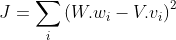# Pytorch crashes on optimizer.step() due to insufficient memory

Hello,

I am trying to calculate loss on a function which looks like this:W is a vector of shape (314287, 300), whereas V is a vector of shape (314287, 3000)… (I am trying to learn a sparse representation).

The relevant portion of my code is as follows:

``````model = torch.optim.Adam([sparse_embeds], lr=1e-4)
embeds = embeds.cuda()
sparse_embeds = sparse_embeds.cuda()
buckets_size = 1000

def bucketize(tensor, buckets):
total_buckets = int(tensor.shape / buckets) + 1
i = 0
while i*buckets < tensor.shape:
yield i*buckets, (i+1)*buckets
i += 1

for e in range(50):
for i in range(len(embeds)):
for se in bucketize(embeds, buckets_size):
start, end = se
all_dots = embeds[start:end] @ embeds[i]
s1 = (sparse_embeds[start:end] @ sparse_embeds[i]) - all_dots
loss = torch.sum((s1.pow(2)))

print(loss.item())
loss.backward()
model.step()   # <<<<<<<<< Memory crashes here.
``````

I had thought that breaking the loss calculation in multiple buckets would have solved the issue but it doesn’t seem to. Any help at all would be appreciated.

Hi,

Have you tried to reduce the embedding size or replace Adam with SGD?
The Adam optimizers will allocate a couple buffers with the same size as the weights to store all the statistics it needs. So that might be too much for your machine?

1 Like

As @albanD suggested try considering SGD instead of Adam as Adam needs more memory for storing first and second moment vectors.

Also, reducing embedding size and bucket_size and overall architecture might help.
Also want to suggest you that, `bucketize()` only needs tensor_shape instead of the whole tensor.

1 Like

Hey @albanD, I am trying out SGD and it is working. Thank you for the suggestion. A question though. If I am optimizing over a huge tensor with Adam but am only operating on a narrowed view of the tensor at a time. Will the optimization procedure still update (or allocate memory for updating) all the gradients? Can I change this behavior in any way?

I am not very familiar with what it does in details, but I guess that’s what SparseAdam is for: https://pytorch.org/docs/stable/optim.html?highlight=adam#torch.optim.SparseAdamI was not sure if reducing the bucket size helped (I tried with a low value in the first place). Perhaps Adam still updates all tensors (even if they were not involved in gradient computation). Can you suggest me if and how I could change this behavior?

``````RuntimeError: SparseAdam does not support dense gradients, please consider Adam instead
``````

I suspect it only works with sparse tensors. I am actually working with dense tensors (to learn a sparse representation). Regardless, I think I will make do with SGD for now. Thank you again @albanD

I don’t know the exact problem but optimizer updates only those parameters which leads to loss computation by some means and whose `requires_grad` is set to True. Try setting `requires_grad` of the parameters you don’t want to update, to False or even `detach()` those parameters from backward computation graph if you also want layers below it not to be updated.